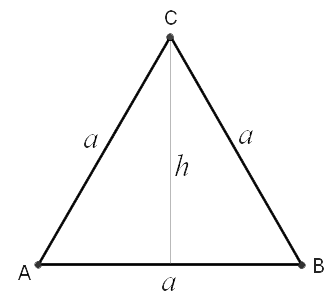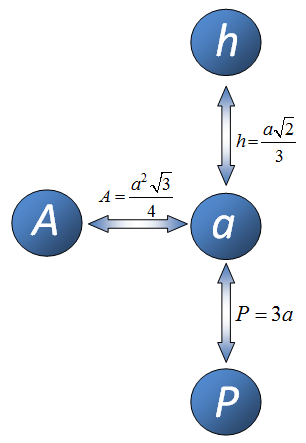Math Calculators, Lessons and Formulas

It is time to solve your math problem

mathportal.org

# Equilateral Triangle Calculator

Enter side, perimeter, area or altitude of equilateral triangle then choose a missing value and the calculator will show you a step by step explanation how to find that value.

Equilateral Triangle Calculator
Input value you know and select what to compute.
show help ↓↓ examples ↓↓
I want to calculate:
Input one element of a triangle
use letter r to input square root. Ex: $3\sqrt{2} = \text{3r2}$
side $a$
=area $A$
=
perimeter $P$
=
height $h$
=

working...
examples
example 1:ex 1:
What is the area of an equilateral triangle of perimeter $90 cm^2$.
example 2:ex 2:
What is the perimeter of an equilateral triangle if its height is $\frac{20}{3} cm^2$?
example 3:ex 3:
If base of an equilateral triangle $50$ inches long, what is the triangle's height?
example 4:ex 4:
$\triangle ABC$ is an equilateral triangle with area 24. Find the perimeter of $\triangle ABC$.

# Formulas for Equilateral Triangle

To calculate missing value in equilateral triangle, based on one known value, you need to remember just three formulas.F1: $$P = 3 \cdot a$$ a = side h = altitude A = area P = perimeter F2: $$A = \frac{a^2 \sqrt{3}}{4}$$ F3: $$h = \frac{a \sqrt{3}}{2}$$

The diagram at the right shows when to use each of these formulas.

## Example 1:

If you are given altitude h and you want to calculate side a, then you need to use formula which connects h and a.

## Example 2:

If you are given area A and you want to calculate perimeter P then you need to make two steps to get the solution. In first step you apply formula which connects area A and side a and in second step use formula which connects side a and perimeter P.

Quick Calculator Search